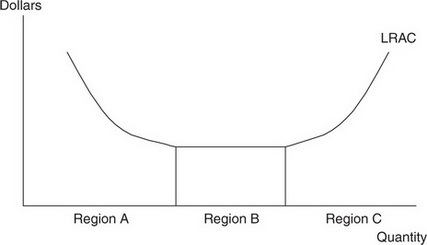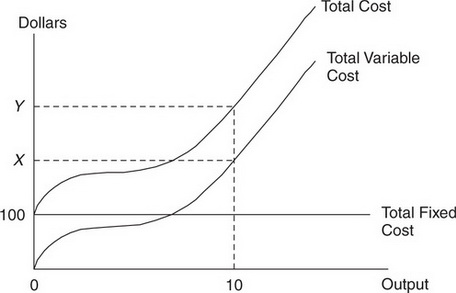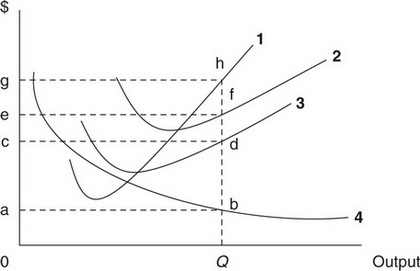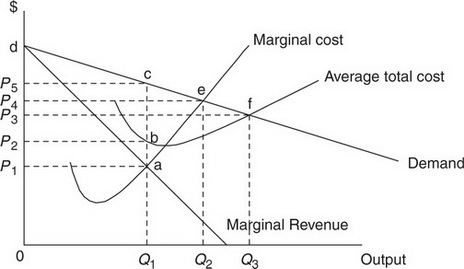# AP Microeconomics Practice Test 21

### Test Information15 questions18 minutes

1.The figure above shows the long-run average cost curve of a competitive firm. Which of the following choices best describes Region B in the diagram?

2. The market for good X is currently in equilibrium. Which of the following choices would not cause both a decrease in the equilibrium price of good X and a decrease in the equilibrium quantity of good X?

3. The figure below shows cost curves for a competitive firm.If average variable cost at a quantity of 10 is \$25, what is the value of \$Y in the figure above?

4. The figure below shows cost curves for a competitive firm.At a quantity of 10, what is the value of \$(Y - X )?

5. The demand for labor falls if

6.The curve labeled 4 represents which of the following?

7.Where is the shutdown point for this perfectly competitive firm?

8. If a market for a good is producing a negative externality,

9. Which of the following is a characteristic of a monopoly market?

10. A monopolist may be able to maintain long-run positive profit due to

11.If this firm were a profit-maximizing monopolist, the price, output, and profit would be

PRICE     OUTPUT     PROFIT

12.Consumer surplus in the monopolist market is equal to the area

13. The top six firms in an oligopolistic industry have market shares of 25%, 25%, 15%, 10%, 6%, and 3%. Many smaller firms split the rest of the market. What is the value of the four-firm concentration ratio?

14. Which of the following statements is true of a consumer's utility-maximizing behavior?

15. Oligopoly has at times been the subject of government antitrust regulation. Which of the following is a reason for this government regulation?